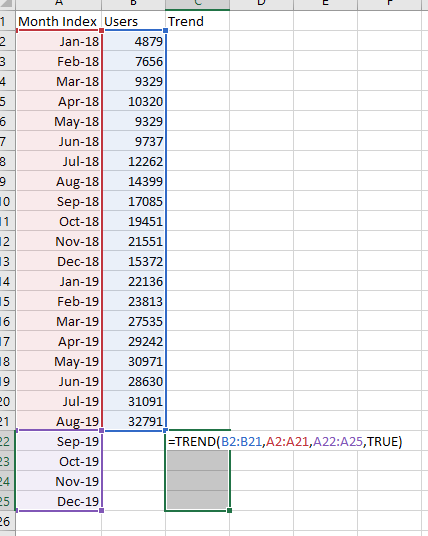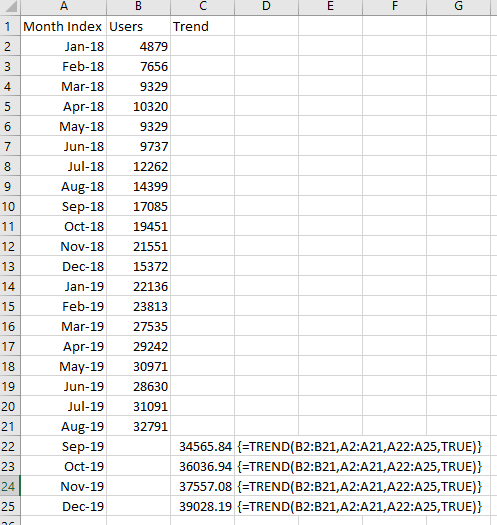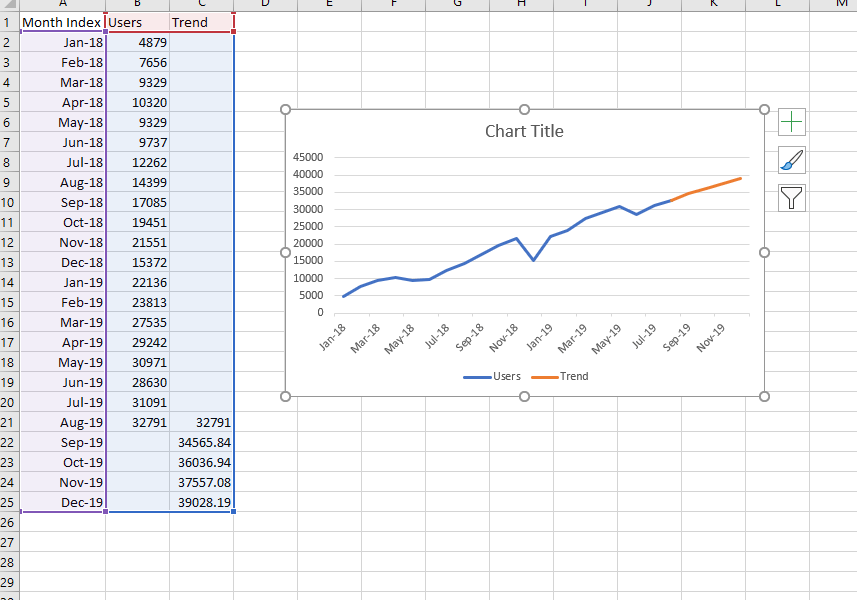# Easy Forecasting in Excel

Forecasting with the Trend line

1. Click on your line graph.
2. Click the [ + ] button on the chart to open the chart elements menu.​
3. Choose the trend line (Linear or Exponential).
4. Right Click the Trend line, Choose Format Trend line.
5. Under Forecast choose the number of the period into the future you want to forecast.

Use Array Functions (Growth and Trend Functions)

Array functions are calculations that can be performed on an array of values. You can think about these as a list of values. This performs the same function as above however; this will provide you with the values in your table.   Both the GROWTH() and TREND() will be using your y values to predict

1.  Increase your date to the future number in this case, we are extending 3 extra periods into the future.
3. Highlight all your new dates.
4. Enter the TREND function while the new dates are highlighted. Only the first cell will have the formula.. This function takes in all xs in your data.
5.   Enter the parts of the formula
• Present Dates represents the known_xs,
• Present Values represents known_ys.
• New xs represent the future dates
• True uses the intercept which produces better accuracy.
6. Press Ctrl-Shift-Enter to enter the formula in the new dates.Press Ctrl-Shift-Enter to fill the new cells.Add a chart. However to fill in the missing whole just copy the last month into the trend column.  The trend is an orange.Moving Average

The simplest and easy way to perform a forecast is to use a moving average (MA). This is as simple as it sounds you will user average of a set number of months and use the average to predict X number of periods in the future.

1.  If you are in the first period of your data highlight the first 3 time periods. So, you will be starting your average at the 4th row of your data.
2. Use the AVERAGE function with the first 3 periods of your data. i.e) =AVERAGE (B1:B3).
3.  Drag the formula down.
4.  Create 3 extra time period by dragging the formula 3 spaces below your original data. You have a 3-month MA forecast

Linear Regression

Regression allows drawing the line of “best fit” through your observed data.  This is the same process as the first trend. This line of best fit is the result of the slope and intercept of the data plotted on our y and x axis.

You can get this formula for linear regression from the line example in the beginning.

For this method you will need an index column. This column just counts each row, i.e) 1,2,3….Get the regression formula from the trend line(y=mx+b)

1. Create the Index Column
2. Use the FORECAST.LINEAR(x,known_ys,known_xs)
• x is the new index for the new dates
• known_xs are your index values
3. Use this equation to forecast future dates.#### Gaelim Holland

Subscribe
Notify of1 Comment
Inline Feedbacks